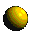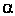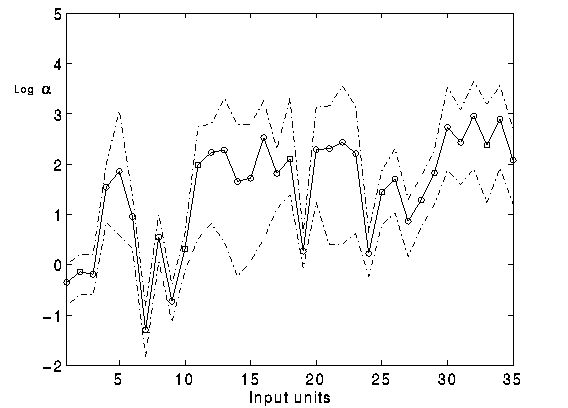Validation and Verification of Neural Network Systems

Automatic Relevance Determination

The investigation of the redundant components of the feature vector can be addressed by the Automatic Relevance Determination method. This technique (proposed by R. Neal and D. MacKay) is able to detect the relevant components of the input vector: this can be achieved by looking at the distribution of the synaptic weights which connect one input unit to all of the units in the next layer. Actually the variance of this distribution can give an idea about size of the weights controlled by each one of the input units.

Namely:A small variance suggests that the weights are quite close to 0: thus the input controlling those weights is not very relevant.Conversely a large variance is typical of distribution of weights which are connected to a relevant input.

In our experiments we controlled the variance of the distribution of weights through a hyperparameter, whereis inversely proportional to the square root of the actual variance. The lower thethe larger the variance.The plot shows the relevance of the input features. The input features are reported on the x-axis;
the y-axis reports the inverse of the variance of the distribution of the synaptic weights
which connect one input unit to all of the units in the next layer.

The experiments we run showed that features describing colour, size and position of the regions are the most relevant in training the neural network, as the variances corresponding to those features have had large values.

All of the experiments have shown that the coordinates (x,y) of the regions have a different weight in training the networks. As the images are taken with the y axis closely aligned to the vertical, the classification of the regions is unlikely to depend upon the x coordinate.

The results presented in this web page have been published in the paper Using Bayesian Neural Networks to Classify segmented Images which is available as compressed postscript .

Contact names

Francesco Vivarelli
Dr. Christopher K. I. Williams
Dr. W. Andrew Wright